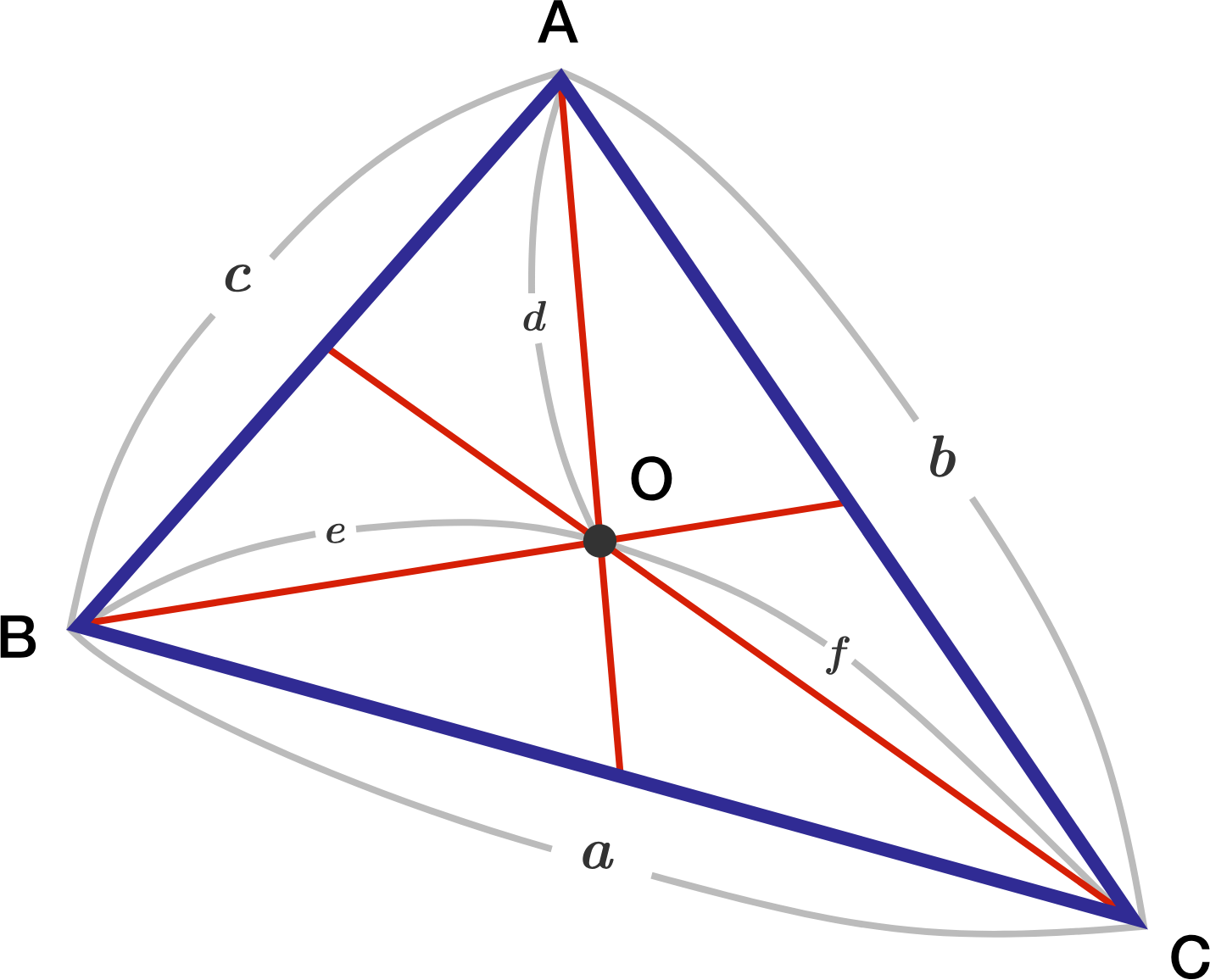# Triangle CentroidLet $a, b, c$ be the side lengths of triangle $ABC$ above, and let $d, e, f$ be the distances from its centroid $O$ to the vertices. (The red lines are the medians.)

What is the ratio $\dfrac{a^2+b^2+c^2}{d^2+e^2+f^2}?$

×